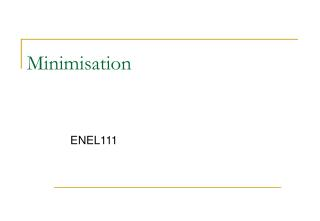DownloadDownload PresentationMinimisation

# Minimisation

Télécharger la présentation## Minimisation

- - - - - - - - - - - - - - - - - - - - - - - - - - - E N D - - - - - - - - - - - - - - - - - - - - - - - - - - -
##### Presentation Transcript

1. Minimisation ENEL111

2. Minimisation • Last Lecture • Sum of products • Boolean algebra • This Lecture • Karnaugh maps • Some more examples of algebra and truth tables

3. Karnaugh Maps • K-Maps are a convenient way to simplify Boolean Expressions. • They can be used for up to 4 or 5 variables. • They are a visual representation of a truth table. • Expression are most commonly expressed in sum of products form.

4. Truth table to K-Map minterms are represented by a 1 in the corresponding location in the K map. The expression is: A.B + A.B + A.B

5. K-Maps • Adjacent 1’s can be “paired off” • Any variable which is both a 1 and a zero in this pairing can be eliminated • Pairs may be adjacent horizontally or vertically a pair B is eliminated, leaving A as the term another pair A is eliminated, leaving B as the term The expression becomes A + B

6. Returning to our car example • Two Variable K-Map A.B.C + A.B.C + A.B.C One square filled in for each minterm. Notice the code sequence: 00 01 11 10 – a Gray code.

7. Grouping the Pairs equates to B.C as A is eliminated. Here, we can “wrap around” and this pair equates to A.C as B is eliminated. Our truth table simplifies to A.C + B.C as before.

8. Groups of 4 Groups of 4 in a block can be used to eliminate two variables: The solution is B because it is a 1 over the whole block (vertical pairs) = BC + BC = B(C + C) = B.

9. Karnaugh Maps • Three Variable K-Map • Extreme ends of same row considered adjacent A BC 00 01 11 10 0 1 10 00

10. Karnaugh Maps • Three Variable K-Map example A BC 00 01 11 10 0 1 X =

11. The Block of 4, again A BC 00 01 11 10 0 1 1 1 1 1 X = C

12. Returning to our car example, once more • Two Variable K-Map A.B.C + A.B.C + A.B.C There is more than one way to label the axes of the K-Map, some views lead to groupings which are easier to see.

13. Don’t Care States • Sometimes in a truth table it does not matter if the output is a zero or a one • Traditionally marked with an x. • We can use these as 1’s if it helps.

14. Karnaugh Maps • Four Variable K-Map • Four corners adjacent AB CD 00 01 11 10 00 01 11 10

15. Karnaugh Maps • Four Variable K-Map example AB CD 00 01 11 10 00 01 11 10 F =

16. Karnaugh Maps • Four Variable K-Map solution AB CD 00 01 11 10 1 00 1 1 01 1 1 11 10 1 1 F = B.D + A.C

17. Product-of-Sums We have populated the maps with 1’s using sum-of-products extracted from the truth table. We can equally well work with the 0’s P = (A + B).(A + C) P = A.B + A.C equivalent

18. Inverted K Maps • In some cases a better simplification can be obtained if the inverse of the output is considered • i.e. group the zeros instead of the ones • particularly when the number and patterns of zeros is simpler than the ones

19. Karnaugh Maps • Example: Z5 of the Seven Segment Display X1 X2 X3 X4 Z5 0 1 2 3 4 5 6 7 8 9 0 0 0 0 1 0 0 0 1 0 X1X2 X3 X4 00 01 11 10 0 0 1 0 1 0 0 1 1 0 00 0 1 0 0 0 01 0 1 0 1 0 11 0 1 1 0 1 0 1 1 1 0 10 1 0 0 0 1 1 0 0 1 0 Z5 = 1 0 1 0 X 1 0 1 1 X • Better to group 1’s or 0’s? 1 1 0 0 X 1 1 0 1 X 1 1 1 0 X 1 1 1 1 X

20. 7-segment display • If there are less 1’s than 0’s it is an easier option: X1X2 X3 X4 00 01 11 10 00 1 0 0 1 01 0 0 0 1 11 X X X X 10 1 0 X X Changing this to 1 gives us the corner group.

21. Tutorial - Friday • Print out the CS1 tutorial questions from the website. • Come to see the answers worked through.